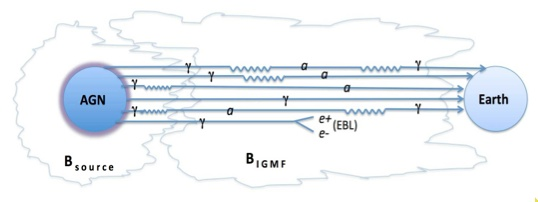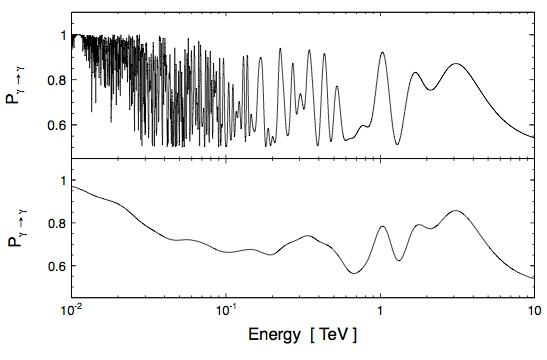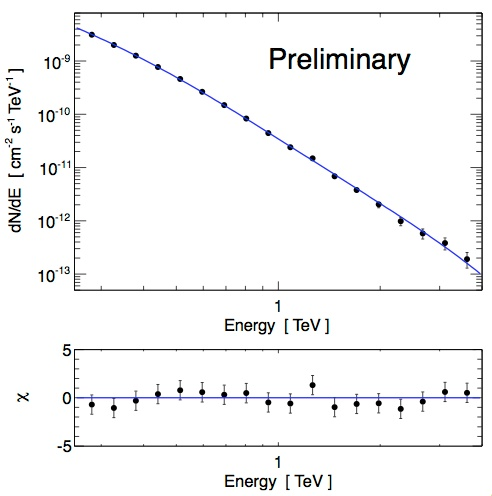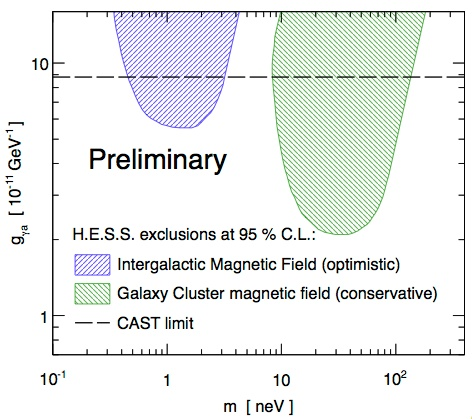# A photon is a photon is an axion is a photon

## August 2013Illustration of gamma-ray propagation in the presence of oscillations between gamma rays and axion-like particles, in the intergalactic magnetic fields. Photon-to-axion oscillations (or vice-versa) are represented by a crooked line, while the symbols 'γ' and 'a' mean gamma-ray photons and axions, respectively. From Sanchez-Conde et al. 2009.

This month's SOM discusses the use of gamma rays from the active galaxy PKS 2155-304 (see SOM Sept. 2007) to search for exotic new particles. Some extensions of the Standard Model of particle physics predict hypothetical new light particles, which interact weakly with matter: axions. In the presence of magnetic fields, photons are predicted to convert into axions and vice versa. In the laboratory, axion searches employ, among other schemes, the "light shining through a wall" technique (e.g. Ehret 2010), where photons in a laser beam immersed in a magnetic field convert to axions, are transmitted through a laser beam stop, and are reconverted into photons detected on the other side of the beam stop, apparently making the laser light shining through the beam stop. So far, axion searches have mostly provided upper limits on the a priori unknown axion-photon coupling strength.

If photons - and hence gamma rays - can indeed convert to axions, this has implications on, and is potentially detectable in the gamma ray spectra of extragalactic sources (e.g. Sanchez-Conde et al. 2009). On their multi-million year journey in the faint intergalactic magnetic fields, gamma rays may oscillate into axions and back, with two consequences (see top illustration): (i) since axions do not interact with the extragalactic background light which limits transparency of the Universe for high energy gamma rays (see e.g. SOM Dec. 2012), the range of gamma rays is larger than it would normally be (e.g. Meyer et al. 2013, Horns et al. 2012), and (ii) since the conversion probability depends on gamma-ray energy, the spectrum of gamma rays detected on Earth is modulated, with a deficit of gamma rays at energies where conversion into axions peaks for a given source distance and magnetic field strength (Fig. 1).

This modulation of spectra was explored using H.E.S.S.' high-statistics gamma-ray spectra of PKS 2155-304 (Fig. 2). Within statistics, spectra appear smooth, allowing to place exclusion limits on the combination of axion mass and photon-axion coupling (Fig. 3). In certain parameter ranges, the limits obtained by this technique provide the most stringent restrictions on the coupling between photons and axions - a very interesting result and promising perspective for future studies with instruments such as the planned Cherenkov Telescope Array CTA.

Reference: H.E.S.S. Collaboration, P. Brun, D. Wouters et al., "Constraints on axion-like particles with H.E.S.S. from observations of PKS 2155-304" (arXiv:1307.6068)Fig. 1: Probability that a gamma ray from the active galaxy PKS 2155-304 is detected as a gamma ray, for a specific choice of axion parameters. The top plot shows the probability as a function of true gamma-ray energy, the bottom plot as a function of measured energy; in the latter, the finite energy resolution of the H.E.S.S. instrument smears out the rapid oscillations for low energy gamma rays.Fig. 2: Measured spectrum of gamma rays from the active galaxy PKS 2155-304. Bottom: relative deviations from a smooth curve.Fig. 3: Exclusion limits in the domain of axion mass (in nano-eV) and axion-photon coupling strength. The shaded regions are excluded by H.E.S.S.; the exact exclusion area depends on the assumptions regarding extragalactic magnetic fields. The dashed line shows the lower limit on axion-photon coupling obtained by the CAST experiment.